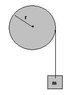# Rotational Dynamics Question

• magnummd

## Homework Statement

Problem: A massless string is wrapped around a solid cylinder as shown in the diagram at the right. A block of mass m = 2.0 kg hangs from the string. When released, the block falls a distance of 82 cm in 2.0 s. Starting with a free-body diagram, calculate the mass of the cylinder.

## Homework Equations

d = Vot + 0.5at^2
Tension = ma - mg
Torque = (I)(alpha) = F(r)

## The Attempt at a Solution

Conceptually, I figure I need to find the force being exerted on the wheel, and the acceleration. That will leave nothing but the mass to be determined. But the fact that it's rotational, throws a wrench into it, especially since no radius is provided.

using d = Vot + 0.5at^2 we get a = 0.41 m/s^2 for the block.
Tension is therefore, 18.78 N in the opposite direction of the block's movement and is the force being exerted on the wheel (I think).

Torque = 18.78(r) = 0.5Mr^2(a/r)

If my approach is right, I need M, but r is in the way. What to do?

Last edited:

Torque = 18.78(r) = 0.5Mr^2(a/r)

If my approach is right, I need M, but r is in the way. What to do?

Wait a minute... the radii all cancel... therefore M = 37.56/0.41 = 91.6 kg

Did I figure it out, or did I just dig myself into a deeper hole? Help please!

I don't really know where I could attach a photo of it, but it essentially looks like a string hanging off a pulley with a mass on the end.

Any suggestions on how I can show it to you?

Last edited:
When you make a new reply below "submit reply" look for a button called "Manage Attachments".

Sweet thanks! Here it is:

#### Attachments

•Assignment Question Diagram.JPG
2.5 KB · Views: 589
I'm new to this forum but just a general hint i post my pictures on photobucket.com and then just click the image button where i want it in the post and paste in the link ...